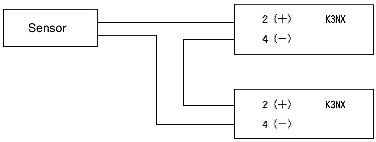# Can one sensor with a 4- to 20-mA output be connected to two K3NX or K3TS Digital Panel Indicators?

It is possible to connect two Meters. Connect the Meters in parallel, as shown in the following diagram.Note:
The input resistance of the K3NX and K3TS is 10 Ω. When connecting two K3NX or K3TS Process Meters, the sensor's permissible load resistance must be 20 Ω or higher.

Calculating the Maximum Number of Connectable Meters:

Use the following equation to determine how many Meters can be connected.

Sensor's permissible load resistance > Digital Panel Meter's input resistance × Number of Meters